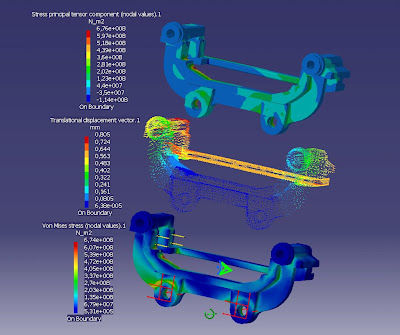tisdag 29 mars 2011

Caliper bracket analysisAs you can see, I put my bracket through the FE analysis of my CAD package. The intention was to get loads to use with the adapter.

So what did I put into the analysis then? Well, for restraints I used the normal type of constraint. The two faces which are in contact with the adapter were locked from sliding along the adapter. On the opposite side, the edge of the hole was locked from moving perpendicularly to the bracket. I realize now that this version is not entirely true as the bolt is threaded into this hole in the bracket. well, I'll see some other day if it makes a difference.

The load was applied where the pad backings make contact with the bracket.

The load was determined by a few calculations. First, I aimed for a stopping distance of 30m (which is unlikely, but something to aim for) which means an average deceleration of 1.3G. I then calculated the balance at this rate of deceleration which gave me the normal forces acting on the wheel. The formula then was:

my x N x D/2 = F x d/2

where "my" is the friction coefficient between tire and ground (1.3 to reach the deceleration rate I aimed at), "N" is the normal force acting on each wheel, "D" is the wheel diameter and "d" is the effective diameter of the rotor. "F" then is the force acting on the bracket.

I calculated F to be about 13.2kN. However, for the analysis I set F to 15kN.

And the end result is the picture at the top.